Courses

# Test: Coordination Compounds- 1

## 30 Questions MCQ Test Chemistry Class 12 | Test: Coordination Compounds- 1

Description
This mock test of Test: Coordination Compounds- 1 for Class 12 helps you for every Class 12 entrance exam. This contains 30 Multiple Choice Questions for Class 12 Test: Coordination Compounds- 1 (mcq) to study with solutions a complete question bank. The solved questions answers in this Test: Coordination Compounds- 1 quiz give you a good mix of easy questions and tough questions. Class 12 students definitely take this Test: Coordination Compounds- 1 exercise for a better result in the exam. You can find other Test: Coordination Compounds- 1 extra questions, long questions & short questions for Class 12 on EduRev as well by searching above.
QUESTION: 1

### The correct IUPAC name of [Fe(C5H5)2] is

Solution:

The correct answer is option B.

The I.U.P.A.C. name for Fe(C5H5)2 is bis(η5 -cyclopentadienyl) iron(II)
The oxidation state of iron is +2 and is written in parenthesis in roman numerals. Two cyclopentadienyl ligands are coordinated to Fe. The prefix bis indicates 2. η5 indicates that the cyclopentadienyl ligands are penta coordinates.

QUESTION: 2

### Type of isomerism exhibited by [Cr(NCS)(NH3)5] [ZnCl4] :

Solution: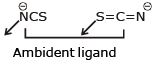Since both cation & anion constitute coordination sphere so it exhibit coordination isomerism and contains ambident ligand so, it shows linkage isomerism

QUESTION: 3

### A complex compound in which the oxidation number of a metal is zero is

Solution:

A complex compound in which the oxidation number of metal is zero is [Ni(CO)4​]. In this complex, the oxidation number of both metal and ligand is zero.

The oxidation number of metal in the complexes K4​[Fe(CN)6​], K3​[Fe(CN)6​] and [Pt(NH3)4]Clare +2, +3 and +2 respectively.

QUESTION: 4

Trioxalato aluminate (III) and tetrafluorido-borate (III) ions are respectively :

Solution:

O. N. of Al = +3
O. N. of B = + 3
[BF4]-
[Al(C2O3)3]3-

QUESTION: 5

Which of the ligand can show linkage isomerism and acts as flexidentate ligand:

Solution: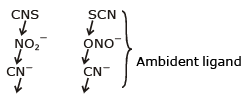QUESTION: 6

Consider the following statements, "According the Werner's theory. :

(1) Ligands are connected to the metal ions by covalent bonds.

(2) Secondary valencies have directional properties.

(3) Secondary valencies are non-ionisable.

(4) Secondary valencies are satisfied by either neutral or negative legands.

Of these statements.

Solution:

Consider werner’s theroy

QUESTION: 7

From the stability constant (hypothetical values), given below, predict which is the strongest ligand:

Solution:

Higher the value of K higher will be strength of ligand & more will be thermodynamic stability of complex produced.

QUESTION: 8

The complexes given below show: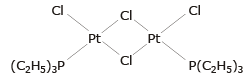and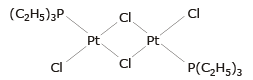Solution: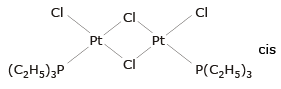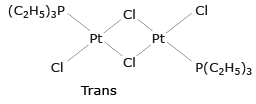QUESTION: 9

In which of the following complexes the nickel metal is in highest oxidation state.

Solution:

[Ni0(CO)4]
[Cr3+(NH3)2][Ni4+F6]3
[Ni2+(NH3)2][B+3F4]2
K4[Ni+2(CN)6]

QUESTION: 10

An ion M2+, forms the complexes [M(H2O)6]2+, [M(en)3]2+ and [MBr6]4-, match the complex with the appropriate colour.

Solution:

Δo value is directly proportional to energy and inversely related to the wavelength. Also, more the ligand is a strong field ligand More will be the value of Δo and lower will be its wavelength. Among the given complexes the strength of ligands are Br− < H2O < en.

Since the emitted colours are red, green, blue the absorbed colours must be green,red,orange. Hence the  compound [M(H2O)6]2+, [M(en)3]2+, [M(Br)6]4− are blue red and green respectively. Hence option B is correct.

QUESTION: 11

For the complex ion dichlorido bis (ethylene diamine) cobalt (III), select the correct statement.

Solution: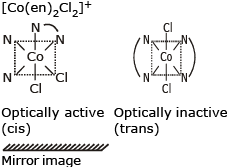QUESTION: 12

Co(CO)4 follows EAN rule by :

Solution:

[Co(CO)4]
EAN = 27 –(0)+ 4 × 2 = 35
2[Co(CO)4] → Co2(CO)8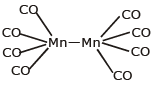EAN = 27 + 1 + 4 ×2 = 36

QUESTION: 13

Type of isomerism exhibited by [Ir(OCN)2(H2O)3]

Solution:

OCN is ambident ligand & it shows linkage isomerism.

QUESTION: 14

Which of the following complex exhibits geometrical isomerism:

Solution:

[PtBrCl NH3 Py] Pt2+ is dsp2 hybridised & hence geometry is square planner & sq. planner complex with four different ligands shows geometrical isomerism.

QUESTION: 15

A complex of platinum, ammonia and chloride produces four ions per molecule in the solution. The structure consistent with the observation is :

Solution: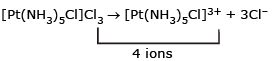[Pt(NH3)4]Cl4 → 5 ions
[Pt(NH3)2Cl4] → 0 ions
[Pt(NH3)4Cl2]Cl2 → 3 ions

QUESTION: 16

The total number of possible isomers of the compound [Cu||(NH3)4] [Pt||Cl4] are :

Solution: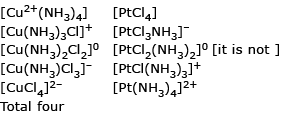QUESTION: 17

In the complex Fe(CO)x, the value of x is :

Solution:

[Fe(CO)x]0
EAN = 26 + 2x = 36
x = 5

QUESTION: 18

The complex which exhibits cis-trans isomerism as well as can be resolved into d and ℓ forms:

Solution: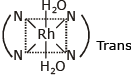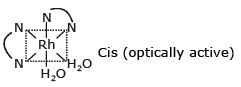QUESTION: 19

The oxidation state of Mo in its oxo-complex species [Mo2O4(C2H4 )2(H2O)2]2- is :

Solution:

Let the O. N. of Mo in the complex is x
2x + (–2) × 2 + 0 × 2 + 0 × 2  = – 2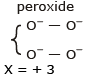QUESTION: 20

The hybridisation and unpaired electrons in [Fe(H2O)6]2+ ion are :

Solution:

H2O is weak field ligand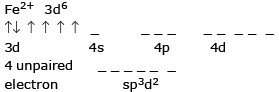QUESTION: 21

In which complex is the transition metal in zero oxidation state:

Solution:

K4[Ni(CN)4] Let O.S of Ni = x
4 + x – 4 = 0
x = 0
(A) Co3+  (B)  Fe2+  (C) Co–1

QUESTION: 22

Formula of ferrocene is :

Solution: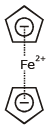QUESTION: 23

The hybrisation of Co in [Co(H2O)6]3+ is :

Solution:

In this complex compound the total charge is +3 as H2O is a neutral compound so the oxidation state of cobalt is +3 and the electronic configuration of Co is 3d7 4s2. So, Co(+3)=4d6 and H2O is a weak ligand so there is no pairing of electron. So,4s 4p3 and 4d2 orbital make hybrid orbital to have a hybridization of sp3d2.

QUESTION: 24

Which of the following is π complex :

Solution:

Al(C2H5)3 σ - complex
Fe(C5H5)2 π - complex
Zn(C2H5)2 σ - complex
[Ni(CO)4] σ - complex

QUESTION: 25

Which complex is likely to show optical activity

Solution:

Cis [Co(NH3)2(en)2]3+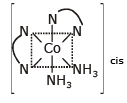No POS optically active rest compound has POS.

QUESTION: 26

Which one is the most likely structure of CrCl3·6H2O if 1/3 of total chlorine of the compound is precipitated by adding AgNO3 to its aqueous solution :

Solution:

Coordination no. of Cr = 6
1/3 × 3 = 1 Chloride ion is outside the coordination sphere.

QUESTION: 27

The two compounds [Co(SO4)(NH3)5]Br and [Co(SO4)(NH3)5]Cl represent :

Solution:

Different molecular mass, so no isomerism

QUESTION: 28

The structure of iron pentacarbonyl is :

Solution:

[Fe(CO)5] TBP
CO is strong field ligand
Fe - 3d64s2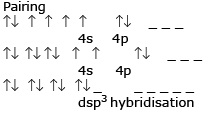QUESTION: 29

The EAN of platinum in potassium hexachloroplatinate (IV) is :

Solution:

K2+4[PtCl6]
At No. Pt = 78
EAN = 78 – 4 + 6 × 2 = 86

QUESTION: 30

Diethylene triamine is :

Solution:Track your progress, build streaks, highlight & save important lessons and more!

### Similar Content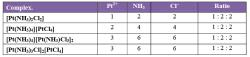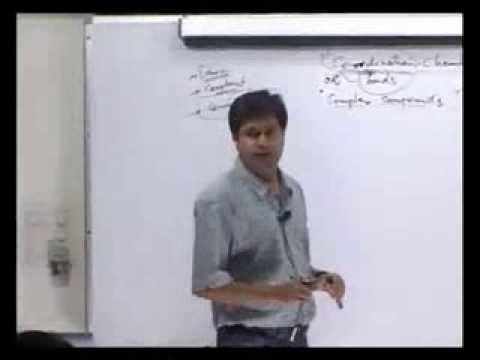### Related tests